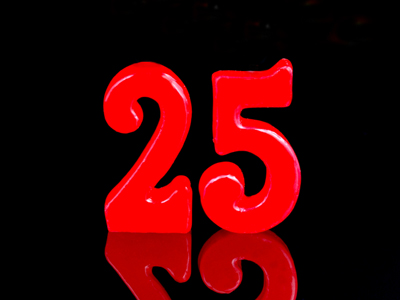If you multiply 5 by itself, you get 25.

# Properties of Numbers (Year 5)

In Year 5, which is the third year in KS2 Maths, you'll learn cool things about numbers. Numbers have special friends called factors and multiples. Imagine factors as numbers that can team up to make another number, like 1, 2, 4, and 8 for the number 8! Multiples are like a group of friends in a times table – for 8, it's 0, 8, 16, and so on. Maths is like solving number puzzles! How much do you know about these number pals?

Let's play a fun quiz for 9-10 year olds and test your knowledge of the properties of numbers. See how many number secrets you can remember!

1.
Which number multiplied by itself gives 49?
3
6
5
7
2.
Which of these numbers is a multiple of 4?
5,698
2,463
4,441
2,548
We can easily work this out as 2,548 is the only number where the last 2 digits are divisible by 4
3.
Which number has the factors of 1, 2, 4, 8 and 16?
8
16
24
36
Every number is a factor of itself as it is itself multiplied by 1
4.
What is a square number?
A number that can be divided by 4
A number that is a multiple of 6 and 7
The product when a number is multiplied by itself
A number that is written in a square
For example 3 x 3 = 9 therefore 9 is the square number of 3
5.
Which of these numbers is a multiple of 7?
15
22
35
37
35 is 5 x 7
6.
What is 5 squared?
5
10
15
25
5 x 5 = 25
7.
What is a factor?
A factor is a whole number that divides into another whole number
A factor is a whole number that can be divided by 6
A factor is a number that cannot be divided
A factor is the product when a number is multiplied by itself
For example, 3 and 2 are both factors of 6
8.
How many pairs of factors will give 12?
1
3
6
12
They are 1 x 12, 2 x 6 and 3 x 4
9.
What is the square number of 6?
6
12
36
60
36 is 6 x 6
10.
How do we know that a number is a multiple of 4?
It ends in 2, 4 or 8
The last two digits are divisible by 4
It is also a multiple of 8
There is no way of knowing
We can ignore numbers in the 100s column as 100 is itself divisible by 4